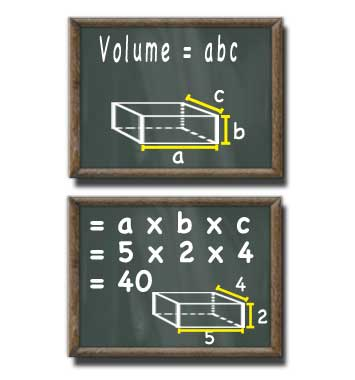# Rectangular Prism Volume Calculator

## Rectangular Prism Volume

#### Volume of a Rectangular Prism with Three Known Sides

Result:
40Width (a) units
Height (b) decimals
Depth (c)

A rectangular prism with a width of 5 units a height of 2 units and a depth of 4 units has a volume of 40 cubic units.

This calculator and more easy to use calculators waiting at www.KylesCalculators.com### Calculating the Volume of a Rectangular Prism:

This formula can be used to calculate to volume of a rectangular prism with known measurements for all three sides:

V = abc

Typically the various sides of this three dimensional shape are labelled a, b and c. If you prefer you can think of these as the width, height and depth. By multiplying all three side lengths together you have the volume of the three dimensional shape.

Below is an example of how to find the volume if three side lengths are known. In the example the rectangular prism is 5 units wide, 2 units tall and 4 units deep:

• V = abc
• = 5 x 2 x 4
• = 40